# Area of a Trapezoid - An Introduction To Geometry

By: Henrique Bertulino

10 min

0

10.04.2022Students have to do different geometric homework. However, the most difficulties arise for middle school students because they studied only mathematics and algebra, and geometry. For example, they need to find the perpendicular distance, surface area, or parallel sides of the trapezoid. Today we will talk specifically about trapezoids, finding the area, and considering it one of the most important theorems.

## Trapezium - What Is This Figure?

A trapezoid is a quadrilateral that has two parallel sides and two non-parallel sides. The parallel sides are called the trapezoid bases, and the other two are the lateral sides. The trapezoid height is the distance between the lines on which the bases of the trapezoid lie, any common perpendicular of these lines. The midsegment of a trapezoid is a segment connecting the midpoints of the sides.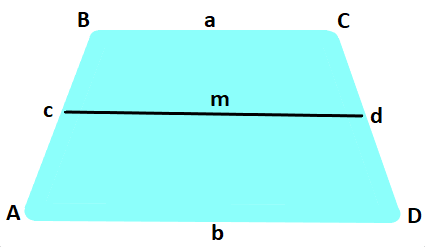## Trapezium Features

If a circle is inscribed in the trapezoid, then the sum of the basics always matches the sum of the sides: a + b = c + d, and the middle line is always equal to half-sum of the sides: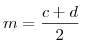An isosceles trapezoid is a trapezoid whose sides are equal to AB = CD. Then the diagonals AC = BD and the angles at the base are equal: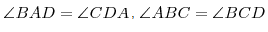Of all the trapezoids, only about an isosceles trapezoid can a circle be described if the sum of the opposite right angles is 180 °. In an isosceles trapezoid, the distance from the top of one base to the opposite top's projection, which is directly connected to the base, is always precisely the centerline.

A rectangular trapezoid is a type of trapezoid that contains a base angle of 90 °.

## Theorem: Area of Trapezoid

To calculate an arbitrary polygon area, we do this: divide the polygon into triangles and find a triangle area. The sum of the areas of these triangles is equal to the area of the polygon. Using this technique, we derive a formula for calculating the area spare the trapezoid. Let us agree to call the height of a trapezoid a perpendicular drawn from any point of one of the bases to a straight line containing another base. In the figure below, we have indicated that the BH line segment is the ABCD trapezoid height: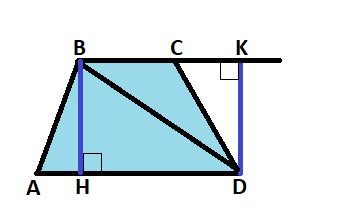Based on this, we get the theorem: "The area of a trapezoid is equal to the product of the half-sum of its bases and the height." Based on the area formula, we can prove this theorem.

The trapezoid given: ABCD, AD, and BC - lengths of the bases, BH - height.

Prove: the area of this trapezoid ABCD will be equal to S = ½ (AD + BC) · BH.

Proof: draw a diagonal BD. It divides the trapezoid into two triangles ABD and BCD. This means that the perimeter of a trapezoid ABCD will be equal to the sum of these triangles' areas.

In a triangle, ABD: AD is the base, and BH is the height. In a triangle, BCD: BC is the base.

Let's draw the height DK. Area S of the triangle ABD = 1/2 AD · BH; area S of the triangle BCD = 1/2 BC · DK. Since BH = DK, then the area S of the triangle BCD = 1/2 BC · BH. Thus, the area S of the trapezium ABCD = 1/2 AD · BH + 1/2 BC · BH = 1/2 (AD + BC) · BH. What was required to prove.

## Calculating Areas in Past Times

Even 4-5 thousand years ago, the Babylonians knew how to determine a trapezoid area in square units. The ancient Egyptians 4,000 years ago used almost the same tricks as we do: the parallel sides' sum was divided in half and multiplied by the height.

Determining the areas of geometric shapes is one of the most ancient practical problems. People did not immediately find the correct approach to their solution. One of the simplest and most affordable ways to calculate areas was discovered by Euclid. When calculating areas, he used a simple technique called the partitioning method.

## Calculating Areas in Modern World

Today there are many formulas for calculating side lengths, vertices, parallel bases, and area trapezoid. We will cover the most basic ones. The formulas below are easy to use, but if you find it challenging to understand and need homework help, you can always contact our service. Experienced authors will advise you on all tasks, and you will significantly improve your academic performance.

### The Formula for Trapezoid Area by Base and Height

Let us be given an arbitrary trapezoid. To find its area, we use the following formula: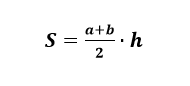In this formula:

• a, b - the base of the trapezoid;
• hh is the height of the trapezoid.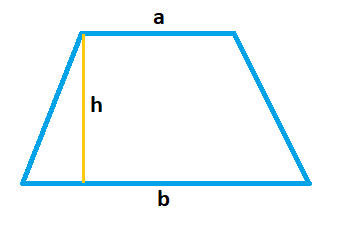Imagine that we need to find a trapezoid area where the bases are known, numerically equal to 10 cm and 8 cm. Also, the well-known height, 6 cm long.

Decision:

• a = 8;
• b = 10;
• h = 6;

We immediately substitute the numbers into the formula we have and calculate the value: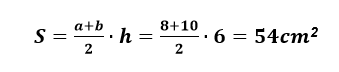### The Formula for the Area of a Trapezoid at the Base and Centerline

We should mention that the middle line of a trapezoid is half the sum of its bases. Thus, finding the area through the centerline is nothing more than a method similar to the first. Insofar as: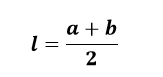In this formula:

• S = l ⋅ h;
• l is the middle line of the trapezoid;
• h is the height.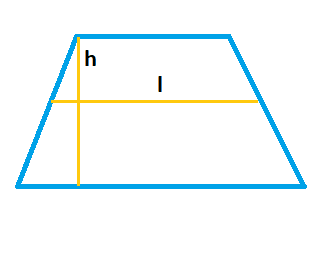Suppose we need to find a trapezoid area if it is known that the middle line is 5 cm, and the height of the trapezoid is two times its height.

Decision:

• l = 5;
• h = 2 ⋅ l.

Find the height of the trapezoid:

h = 2 ⋅ 5 = 10

Area:

S = l ⋅ h = 5 ⋅ 10 = 50 cm.sq.

### The Formula for the Area of a Trapezoid Through the Inscribed Circle Radius and Angle

This case is only suitable for an isosceles trapezoid: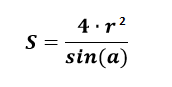In this formula:

• r is the radius of the inscribed circle;
• α is the angle between the base and the side.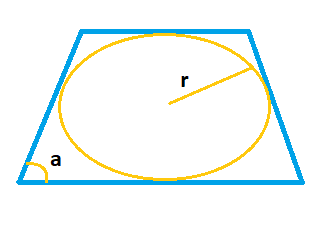Suppose we are given the radius of the inscribed circle in the trapezoid equal to 4 cm. The angle α is equal to 90 degrees. We need to find the area of the trapezoid.

Decision:

• r = 4;
• α = 90.

According to the formula: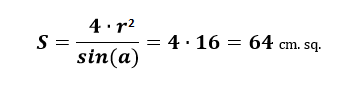### The Formula of the Area of a Trapezoid Through the Diagonals & The Angle Between Them

There is a simple formula for finding the area of a trapezoid through the diagonals and the angle between them: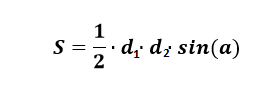In this formula:

• d1, d2 - trapezoid diagonals;
• α is the angle between the diagonals.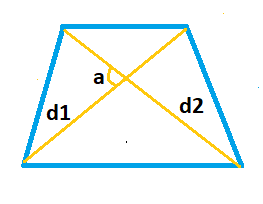Let the two diagonals of the trapezoid be 20 cm and 7 cm. When they intersect, they form an angle of 30 degrees. We need to find the area of the trapezoid.

Decision:

• d1 = 20;
• d2 = 7;
• α = 30 °.

Area: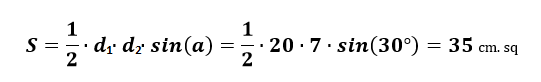## Trapezium And Constellations

The trapezoid is found not only in math homework. This figure can be found when studying the constellations. The spring sky's prominent asterism is the Leo trapezoid, which is observed in the evenings from February to May. This figure is located in the zodiacal constellation Leo, forming an animal's body, and in shape, it resembles a trapezoid.The four bright stars of the constellation α, β, γ, and δ are located at the tops of a trapezoid - the body of a lion. And the head of the lion is formed by stars arranged in the form of a sickle. Hence the name - Leo's trapezoid.

## Trapeziums in Experimental Physics View

The union of physics and mathematics presupposes the continuous movement of science forward. In physics, scientists make experiments, the essence of which becomes fully clear only after mathematical analysis. Many branches of mathematics owe their origin and further development to new physical experiments. As an example, consider school physics laboratory work.

Statement of a question: Let's consider a shape - an arbitrary trapezoid ABCD. Draw two of its diagonals AC and BD, which divide the trapezoid into four triangles - ABO, BCO, CDO, and DAO. Triangles ABO and CDO are equal: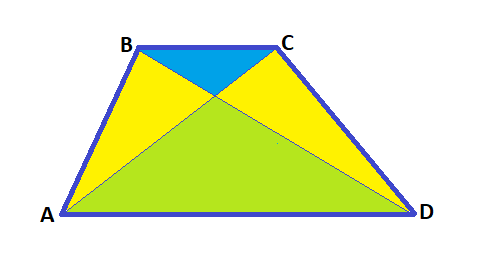The formulation of the goal of the experimental work: using weighing, prove that the masses of the triangles obtained by the diagonals and sides of the trapezoid are equal.

Laboratory work progress:

1. Students need to take: a sheet of paper, a ruler, a pencil, an eraser, scissors.
2. Students draw parallel lines using rectangular rulers.
3. Students need to build five different trapezoids and draw a diagonal.
4. Students use scissors to cut out those triangles in the trapezoid that have one side of the trapezoid.
5. Using a beam balance, students weigh the cut triangles ABO and CDO.
6. Students write down the results obtained in a table and compare.

As you can see, knowledge about the trapezoid will be helpful to you in physics and other sciences.

User ratings:

User ratings is 4.4 stars.Henrique Bertulino